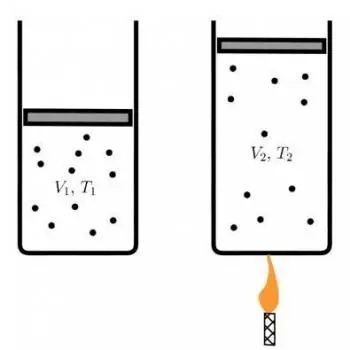# Charles or Volumes Law: Statement, Formula and ExamplesCharles's law, also known as the law of volumes, is one of the fundamental principles in the thermodynamics of gases.

This law, named after the French scientist Jacques Charles, establishes a crucial relationship between the volume of a gas and its temperature in degrees Celsius (°C) at constant pressure.

## Charles' Law Formula

The formulation of Charles' law responds to the following equation:

V/ T1=V/ T2​

Where:

• V 1   is the initial volume of the gas.
• T 1 is the initial temperature in degrees Celsius (°C).
• V 2 is the final volume of the gas.
• T 2 is the final temperature in degrees Celsius (°C).

This equation establishes that the relationship between the volume and temperature of a gas, at constant pressure, is a directly proportional relationship. When the temperature increases, the volume also increases, and when the temperature decreases, the volume of the gas decreases, as long as the pressure remains constant.

## History and Context of the LawCharles's Law is the result of advances in the understanding of gases that took place in the 18th century. Before the formulation of this law, scientists such as Robert Boyle had established the Boyle-Mariotte law, which describes the relationship between the volume and pressure of a gas at constant temperature.

At the same time, Joseph Black had observed that temperature was related to the volume of a gas, although he had not formulated a specific law for this relationship.

The work of Jacques Charles, a French scientist, was essential in cementing this relationship. In 1787, Charles performed a series of experiments that led to the formulation of his law.

His fundamental observation was that, at constant pressure, the volume of a gas increases proportionally to its temperature in degrees Celsius. This means that if the temperature of a gas doubles, its volume also doubles, as long as the pressure remains constant.

## Importance in Gas Thermodynamics

Charles's law is fundamental in gas thermodynamics and has several important implications of which we highlight two:

1. Ideal gas equation: Charles's Law is one of the equations that make up the general ideal gas equation. This equation, PV=nRT, relates the pressure (P), the volume (V), the amount of substance (n) and the temperature (T) of an ideal gas. The relationship between volume and temperature at constant pressure is a key component of this equation.

2. Behavior of gases: The law helps understand how gases behave in response to changes in temperature. This understanding is essential in various applications, such as energy production, industrial chemistry, and engineering.

## Examples of Practical ApplicationsCharles's law has a wide range of practical applications in everyday life and in various industries.

Some examples of these applications include:

• Refrigeration and air conditioning: Refrigeration and air conditioning systems are based on the principle that when a gas is compressed, its temperature increases, and when it is expanded, its temperature decreases. This allows temperature regulation in closed spaces.

• Hot air balloons: Hot air balloons are filled with hot air. When the air in the balloon is heated, its volume increases, allowing it to rise according to Archimedes' principle. Jacques Charles precisely broke a record for flying in a hot air balloon.

• Manufacturing processes: In industry, furnaces and reactors are used such as those used in nuclear power plants that involve water vapor and other gases. Understanding the relationship between volume and temperature is essential in the production of chemicals, metals and other materials.

• Safety in industry: Charles's law is also important in industrial safety. Understanding how temperature changes affect the volume of gases is essential to avoid dangerous situations in chemical plants and industrial processes.

## Conclusion

Charles's law, formulated by Jacques Charles in the 18th century, plays a crucial role in gas thermodynamics. This law establishes a directly proportional relationship between the volume and temperature of a gas at constant pressure.

Its importance is reflected in a wide range of practical applications, from cooling and heating systems to industrial production and gas handling safety.

Charles's work is a testament to the importance of fundamental laws in understanding and applying scientific principles in everyday life and in various industries.

Author:

Published: October 17, 2023
Last review: October 17, 2023